Updating search results...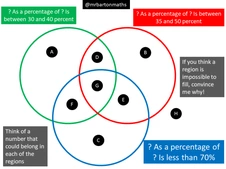# Maths Venns

25 affiliated resources

# Search Resources

View
Selected filters: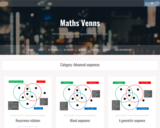Restricted Use
Rating
0.0 stars

These Venn diagrams are like puzzles. Try to find the sequence that means the given restraints. Can you find a sequence that matches all of the restraints and converges or is bounded? Does such a sequence even exist? Use these Venn diagrams to explore sequences in more depth.

Subject:
Mathematics
Material Type:
Activity/Lab
Assessment
Homework/Assignment
Provider:
Maths Venn
Author:
Craig Barton
10/24/2022Restricted Use
Rating
0.0 stars

Have students recognize that an expression can be written in different ways that are equivalent through factoring or multiplying polynomials.

Subject:
Mathematics
Material Type:
Activity/Lab
Assessment
Homework/Assignment
Provider:
Maths Venn
Author:
Craig Barton
10/24/2022Restricted Use
Rating
0.0 stars

This Venn diagram focuses on having students factor and simplify rational expressions.Once students determine one solution to this diagram, have them create their own restrictions for another Venn.

Subject:
Mathematics
Material Type:
Activity/Lab
Assessment
Homework/Assignment
Provider:
Maths Venn
Author:
Craig Barton
10/24/2022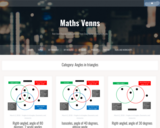Restricted Use
Rating
0.0 stars

Students have been exposed to triangles often throughout the previous years of mathematics. Use these Venn diagrams to have students construct these triangles--if it is possible.

Subject:
Mathematics
Material Type:
Activity/Lab
Assessment
Homework/Assignment
Provider:
Maths Venn
Author:
Craig Barton
10/24/2022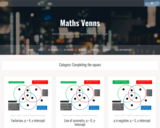Restricted Use
Rating
0.0 stars

These Venn diagrams have students critically thinking about factoring of polynomials, specifically with a focus on completing the square. Determine the restrictions you would like your students to focus on by which Venn diagram you choose.

Subject:
Mathematics
Material Type:
Activity/Lab
Assessment
Homework/Assignment
Provider:
Maths Venn
Author:
Craig Barton
10/24/2022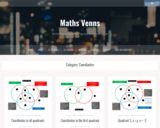Restricted Use
Rating
0.0 stars

Use these Venn diagrams to get students to reason and deepen their understanding of the coordinate plane. Use the Venns that do not focus on quadrant one as extensions for those accelerated students.

Subject:
Mathematics
Material Type:
Activity/Lab
Assessment
Homework/Assignment
Provider:
Maths Venn
Author:
Craig Barton
10/24/2022Restricted Use
Rating
0.0 stars

Subject:
Mathematics
Material Type:
Activity/Lab
Assessment
Homework/Assignment
Provider:
Maths Venn
Author:
Craig Barton
10/24/2022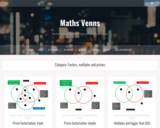Restricted Use
Rating
0.0 stars

Fill in the missing information in the Venn diagram while practicing number sense. Students work with their knowledge of prime numbers, factors and multiples. Can extend to use of exponents.

Subject:
Mathematics
Material Type:
Activity/Lab
Assessment
Homework/Assignment
Provider:
Maths Venn
Author:
Craig Barton
10/24/2022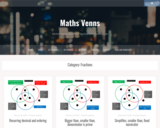Restricted Use
Rating
0.0 stars

Use these Venn diagrams to help support students in learning about fractions. They involve simplifying and comparing fractions with like or unlike denomiators.

Subject:
Mathematics
Material Type:
Activity/Lab
Assessment
Homework/Assignment
Provider:
Maths Venn
Author:
Craig Barton
10/24/2022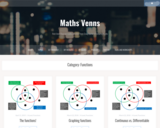Restricted Use
Rating
0.0 stars

Have students think critically about what a function is and their key features through the use of these Venn diagrams. These can be as basic as you would like or extend to Calculus ideas.

Subject:
Mathematics
Material Type:
Activity/Lab
Assessment
Homework/Assignment
Provider:
Maths Venn
Author:
Craig Barton
10/24/2022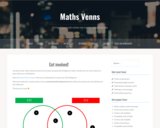Restricted Use
Rating
0.0 stars

If you really like using these Venn diagrams, try creating your own. Or better yet, why not have your students get creative and come up with some venn diagrams that will have them and others thinking deeply about mathematics! This link has a blank template and is a place for you to submit your own creations.

Subject:
Mathematics
Material Type:
Activity/Lab
Assessment
Homework/Assignment
Provider:
Maths Venn
Author:
Craig Barton
10/24/2022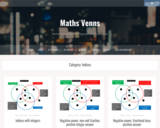Restricted Use
Rating
0.0 stars

Use this collection of Venn diagrams as students are learning the properties of exponents.

Subject:
Mathematics
Material Type:
Activity/Lab
Assessment
Homework/Assignment
Provider:
Maths Venn
Author:
Craig Barton
10/24/2022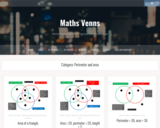Restricted Use
Rating
0.0 stars

Stretch students understanding of perimeter and area through the use of these Venn diagrams. Students try to find the missing information that will fill the requirements for the given area and perimeter problem. If students are just learning area and perimeter, these would work for extension questions for those students who catch on quickly.

Subject:
Mathematics
Material Type:
Activity/Lab
Assessment
Homework/Assignment
Provider:
Maths Venn
Author:
Craig Barton
10/24/2022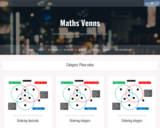Restricted Use
Rating
0.0 stars

Use these Venn diagrams to support student learning of ordering rational numbers with a focus on integers and decimals. Could be extended to numbers written in scientific notation.

Subject:
Mathematics
Material Type:
Activity/Lab
Assessment
Homework/Assignment
Provider:
Maths Venn
Author:
Craig Barton
10/24/2022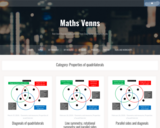Restricted Use
Rating
0.0 stars

How well do your students understand the properties of quadrilaterals and the vocabulary that is used to describe them? Use these Venn diagrams as a way for students to prove which quadrilateral is being described.

Subject:
Mathematics
Material Type:
Activity/Lab
Assessment
Homework/Assignment
Provider:
Maths Venn
Author:
Craig Barton
10/24/2022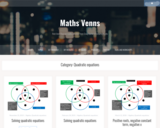Restricted Use
Rating
0.0 stars

These Venn diagrams have students critically thinking about quadratic equations and their structure.

Subject:
Mathematics
Material Type:
Activity/Lab
Assessment
Homework/Assignment
Provider:
Maths Venn
Author:
Craig Barton
10/24/2022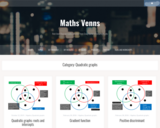Restricted Use
Rating
0.0 stars

Use these Venn diagrams to support students as they continue to deepen their abilities and understanding of quadratic graphs.

Subject:
Mathematics
Material Type:
Activity/Lab
Assessment
Homework/Assignment
Provider:
Maths Venn
Author:
Craig Barton
10/24/2022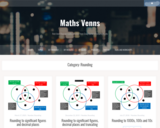Restricted Use
Rating
0.0 stars

Students practice rounding and place value as they work to complete these Venn diagrams.

Subject:
Mathematics
Material Type:
Activity/Lab
Assessment
Homework/Assignment
Provider:
Maths Venn
Author:
Craig Barton
10/24/2022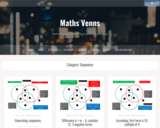Restricted Use
Rating
0.0 stars

Students usually enjoy looking for patterns when it comes to sequences. Have them think differently about a sequence by using the given restrictions on these Venn diagrams.

Subject:
Mathematics
Material Type:
Activity/Lab
Assessment
Homework/Assignment
Provider:
Maths Venn
Author:
Craig Barton
10/24/2022Restricted Use
Rating
0.0 stars

Use this Venn diagram to get students thinking about the different types of symmetry as they use the mathematical practices.

Subject:
Mathematics
Material Type:
Activity/Lab
Assessment
Homework/Assignment
Provider:
Maths Venn
Author:
Craig Barton